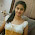## Wednesday, March 12, 2014

### Simple Electronic Circuit Schematic 100 Watts Inverter uisng IC CD4047 and MOSFET IRF540

Robometricschool. - Maybe you will not foreign again with the word INVERTER that you find in electric/electrical field. Inverter used to change DC voltage with low voltage into AC voltage with high voltage.

We think there are many Inverter product that try offer you many kinds of inverter with many powers capacity. But you also can make by your self the simple inverter that can produce enough power to be used charge your device.

In here we will give you one of kinds simple Electronic Circuit Schematic 100 Watts Inverter uisng IC CD4047 and MOSFET IRF540. This circuit schematic can help you to provide output power 100 watts.

Circuit SchematicFigure 1. 100 Watts Inverter (Circuitstoday)

Component Lists
1. C2 = 0.01uF
2. C3 = 2200uF/25V
3. C4 = 0.1uF
4. R2 =  1K
5. R3 = 220
6. R4 = 220
7. R5 = 390K
8. R6 = 1K
9. IC1 = CD4047
10. Q1, Q2 = IRF540
11. T1 = Transformer

Description
1. This circuit schematic using battery 12 Volt 7AH that used as input power DC
2. To generate frequency used IC CD4047 that regulated from R5,R6 and C2
3. Frequency output from IC CD4047 drive MOSFET IRF540 as the power MOSFET
4. Output MOSFET will connected to Transformer T1 as step-up transformer to produce 220V AC with 100 watts.
5. This Circuit schematic will invert 12 VDC from battery into 220 VAC with 100 watt.

1.